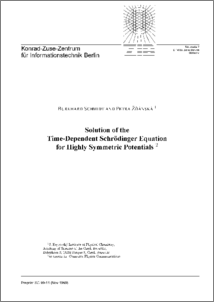Repository: Freie Universität Berlin, Math Department

# Solution of the Time-Dependent Schrödinger Equation for Highly Symmetric Potentials

Schmidt, B. and Zdanska, P. (2000) Solution of the Time-Dependent Schrödinger Equation for Highly Symmetric Potentials. Comp. Phys. Comm., 127 (2-3). pp. 290-308.Preview
PDF - Accepted Version
Available under License Creative Commons Attribution Non-commercial.

2MB

Official URL: http://dx.doi.org/10.1016/S0010-4655(99)00524-X

## Abstract

The method of symmetry adapted wavepackets (SAWP) to solve the time-dependent Schrödinger equation for a highly symmetric potential energy surface is introduced. The angular dependence of a quantum-mechanical wavepacket is expanded in spherical harmonics where the number of close-coupled equations for the corresponding radial functions can be efficiently reduced by symmetry adaption of the rotational basis using the SAWP approach. Various techniques to generate symmetry adapted spherical harmonics (SASHs) for the point groups of highest symmetry (octahedral, icosahedral) are discussed. The standard projection operator technique involves the use of Wigner rotation matrices. Two methods to circumvent numerical instabilities occuring for large azimuthal quantum numbers are suggested. The first is based on a numerical scheme which employs Gaussian integrations yielding exact and stable results. The second is a recursive algorithm to generate higher order SASHs accurately and efficiently from lower order ones. The paper gives a complete set of ``seed functions'' generated by projection techniques which can be used to obtain SASHs for all irreducible representations of the octahedral and icosahedral point groups recursively.

Item Type: Article Mathematical and Computer Sciences > Mathematics Department of Mathematics and Computer Science > Institute of MathematicsDepartment of Mathematics and Computer Science > Institute of Mathematics > BioComputing Group 85 Admin Administrator 03 Jan 2009 20:20 03 Mar 2017 14:39

Repository Staff Only: item control page